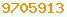subtraction : Java Glossary

subtraction
- is the Java subtraction operator. There are two subtraction operators, integer and floating point, but they both use the same operator symbol. Integer subtraction works on byte, char, short, int and long and always results in an integer, i.e. no fraction. If the results of subtracting two ints is too big to fit in an int, the high order bits will be discarded or considered as a sign bit, without any sort of exception.

Floating point subtraction works on float and double. Floating point subtraction results in a fractional value that can be represented in IEEE (Institute of Electrical & Electronics Engineers) format.

Subtraction is left associative. That means a - b - c means (a - b) - c not a - ( b - c ). Subtractions are performed left to right.

- can also be used an a unary operator, e.g. a = -b;

Learning MoreThis page is postedon the web at: http://mindprod.com/jgloss/subtraction.html Optional Replicator mirror of mindprod.com on local hard disk J: J:\mindprod\jgloss\subtraction.htmlPlease read the feedback from other visitors, or send your own feedback about the site.Contact Roedy. Please feel free to link to this page without explicit permission. Canadian Mind Products IP:[65.110.21.43] Your face IP:[54.144.75.212] Feedback You are visitor number